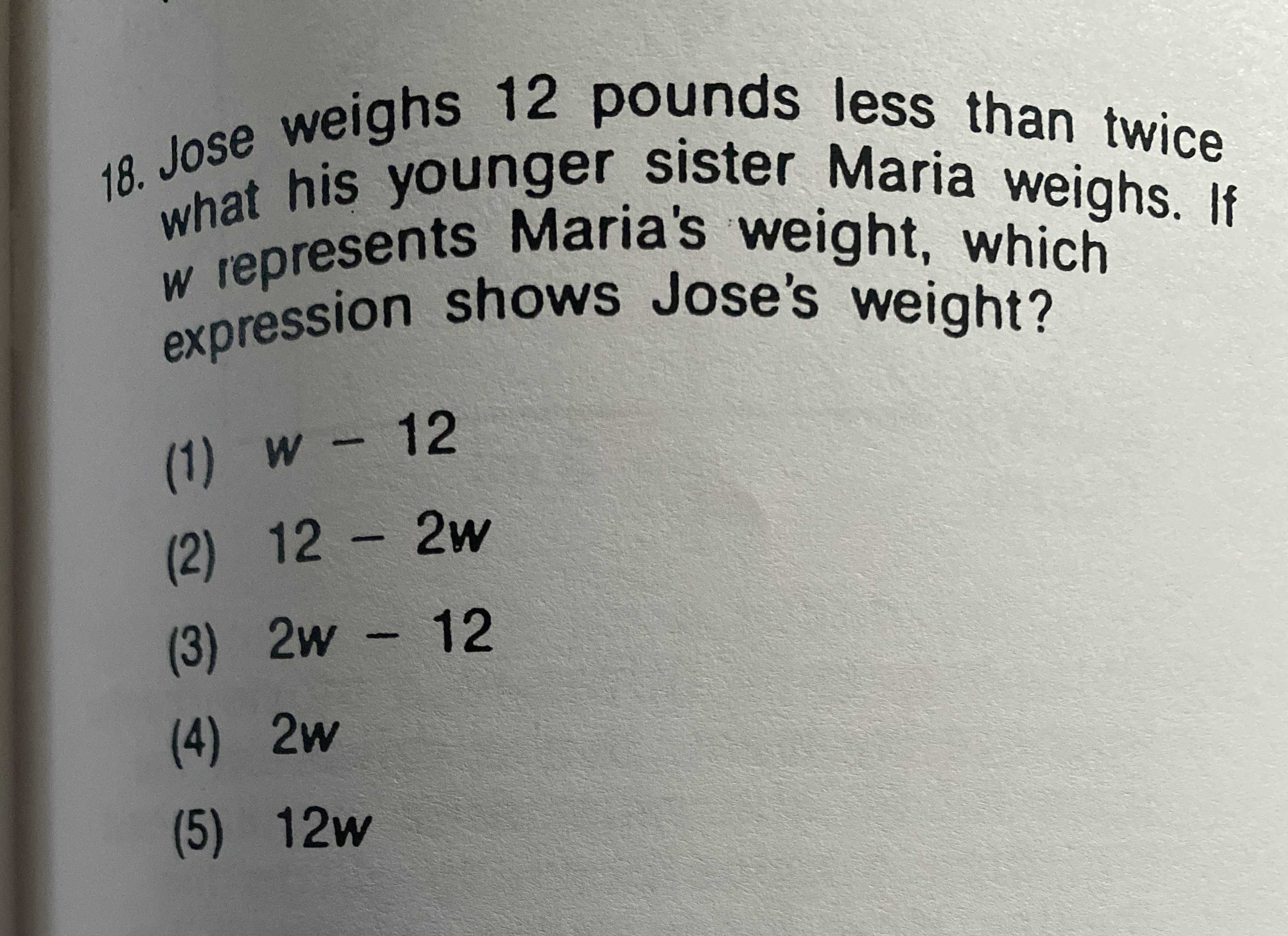### ¿Todavía tienes preguntas de matemáticas?

Pregunte a nuestros tutores expertos
Algebra
Pregunta18. Jose weighs $$12$$ pounds less than twice what his younger sister Maria weighs. If $$w$$ represents Maria's weight, which expression shows Jose's weight?

(1) $$w - 12$$

(2) $$12 - 2 w$$

(3) $$2 w - 12$$

(4) $$2 w$$

(5) $$12 w$$